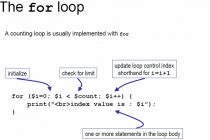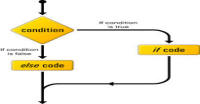# Loops in PHP

Go back to previous tutorial

Show all tutorials on this series till now

Hello friends,

In this article, we will study about loops in PHP. Loops are the repetition of the code. For example, if I want to print 'harish' ten times then I will have to write echo command 10 times, but using loop I will write it only once and it will repeat to print 10 times.

PHP has four types of loops:

1. while loop
2. do while loop
3. for loop
4. foreach loop

In this article, we will study about first three loops because fourth one is dependent upon array which we will learn later. So, we will learn about foreach loop later.

### while loop:

In this loop, we define a initial condition and a terminating condition. The loop starts with initial condition and ends at terminating condition. The syntax of while loop is :

while(condition){.........code.........}

This condition is same as condition of if else statement. While condition is true or non-zero this code will run again and again.

Look at this example:

```<?php

// we are giving an inital value
\$initial_value = 0;

// 10 is terminating condition for loop
while(\$initial_value < 10){
// it's equivalent to echo "harish";echo '
';
echo "harish".'
';
// we are increasing \$initial_value by 1
\$initial_value++;
}
?>
```

In this code, \$initial_value starts from 0 and the loop will run until \$initial value is less than 10. \$initial value is increasing 1 unit per repetition. This code will print 'harish' 10 times. 'br' is used for new line (for printing in different lines). The dot ('.') joins two printing values.

### do while loop:

This loop is also same as while loop but only difference is that it checks condition later and runs code first. In other words, it checks condition only for repetition. Its syntax is:

do{.....code.......} while (condition);

For example:

```<?php

// we are giving an inital value
\$initial_value = 0;

// 10 is terminating condition for loop
do{
echo "harish".'
';
// we are increasing \$initial_value by 1
\$initial_value++;
}while(\$initial_value < 10);
?>
```

This code is also printing 'harish ' ten times. The different occurs only when I initialized \$initial_value with 10 or more value. In this case, while loop will not run but do while will. So, do while loop runs at least one time. Note the semicolon after while command in do while loop.

### for loop:

The syntax of for loop is:

for( initialize, condition, operation){ ....... code ....... }

Before discussing anything first take an example:

```<?php

// initializing inside the loop
for(\$initial = 0; \$initial <10; \$initial++){
echo "harish".'
';
}
// initialize outside the loop
\$initial_value = 0;
for (; \$initial_value < 10; \$initial_value++) {
echo "harish".'
';
}
?>
```

In first two types of loops, we see that first we have to initialize a variable, then we have to check a condition for loop and at last we have to change the value of variable (increment or decrement). In for loop, we do all three things at same place. The for loop takes three arguments:

1. initial value: This value is same as it is for first two types. In for loop, we can initialize a variable in two ways, one inside the loop (in brackets) and another before the loop. Note the syntax in above example. Each argument is separated by semicolon. If you are initializing value outside the for loop then also we have to put semicolon for empty value at initializing place.
2. condition: condition is same as first two cases. It compares the initialized variable value and the given value.
3. operation: operation is also same as before. It changes the value of variable after finishing code of loop each time.

### More examples:

In programming just syntax is not enough to understand the language. Now try understand these examples:

```<?php
//printing number 10 to 0
for (\$number=10; \$number >= 0; \$number--) {
echo \$number.'
';
}
?>
```
```<?php
//increasing number 2 every time
for (\$number=0; \$number < 10; \$number+=2) {
echo \$number.'
';
}
?>
```

In above example, '+=' is an operator which adds right side value to left side value. Try these operators also:

'*=' (multiply like \$number*=2), '-=' (subtraction), '/=' (divide), '%' (modulus or reminder) and '^=' (power).

Now we are writing a program to printing the multiple of 5 between 1 and 100. '%' returns reminder.

```?php
// program to print multiple of 5 between 1 and 100
for (\$number=1; \$number < 100; \$number++) {
if (\$number % 5 == 0) {
echo \$number.'
';
}
}
?>
```

NOTE: We are not taking any tough example here because we will take them in advance series. It is basic series for beginners.

In next tutorial we will study about strings and scopes of variables in PHP. If you like it then please share it with your friend otherwise give your valuable feedback to improve this as well as future tutorial to help other people.

Don't forget to Subscribe for latest feeds.

Next tutorial

#### Click on banner below to Learn: PHP with Laravel for beginners - Become a Master in LaravelHarish, a technical core team member at www.lyflink.com with five year experience in full stack web and mobile development, spends most of his time on coding, reading, analysing and curiously following businesses environments. He is a non-graduate alumni from IIT Roorkee, Computer Science and frequently writes on both technical and business topics.

### Related ArticlesPHP stands for PHP: Hypertext Preprocessor. PHP is known as a server side scripting and interpreter language for web ...
##### Introduction to PHPIn this tutorial, we will study about conditional statements (if else statements and switch statements). These statem...
##### Conditional statements in phpIn this tutorial, we will study about strings and operations upon strings. Due to long length of this section, I have...

### The Complete Web Developer Course - Build 25 Websites